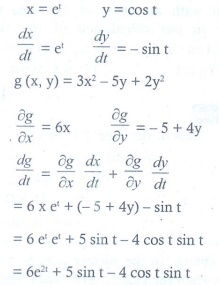# Chapter 8: Differentials and Partial Derivatives - Online Test

Q1. A circular template has a radius of 10 cm. The measurement of radius has an approximate error of 0.02 cm. Then the percentage error in calculating area of this template is
Explaination / Solution: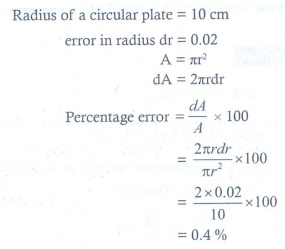Q2. The percentage error of fifth root of 31 is approximately how many times the percentage error in 31?
Explaination / Solution: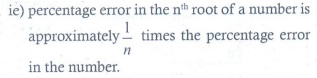Q3. If u ( x, y ) = ex2+y2 , then ∂u/∂x is equal to
Explaination / Solution: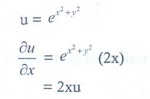Q4. If v ( x, y ) = log(ex + ey ) , then ∂v/∂x + ∂y/∂v is equal to
Explaination / Solution: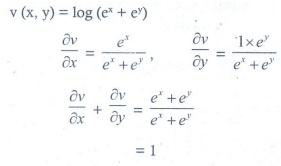Q5. If w( x, y ) = xy , x > 0 , then ∂w/∂x is equal to
Explaination / Solution: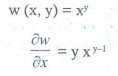Q6. If f ( x, y ) = exy, then ∂2f / ∂x∂y is equal to
Explaination / Solution: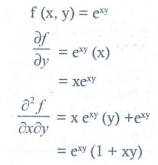Q7. If we measure the side of a cube to be 4 cm with an error of 0.1 cm, then the error in our calculation of the volume is
Explaination / Solution: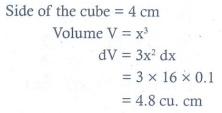Q8. The change in the surface area S = 6x2 of a cube when the edge length varies from x0 to x0 + dx is
Explaination / Solution: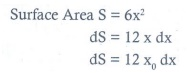Q9. The approximate change in the volume V of a cube of side x metres caused by increasing the side by 1% is
Explaination / Solution: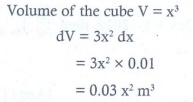Q10. If g ( x, y ) = 3x2 − 5 y + 2 y2 , x (t) = et and y (t) = cos t , then dg/dt is equal to
Explaination / Solution: